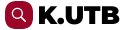TBU Publications
Repository of TBU Publications

# Asymptotic formulas for non-oscillatory solutions of perturbed half-linear Euler equation

## DSpace Repository

﻿
 dc.title Asymptotic formulas for non-oscillatory solutions of perturbed half-linear Euler equation en dc.contributor.author Pátíková, Zuzana dc.relation.ispartof Nonlinear Analysis-Theory Methods & Applications dc.identifier.issn 0362-546X Scopus Sources, Sherpa/RoMEO, JCR dc.date.issued 2008-11-15 utb.relation.volume 69 utb.relation.issue 10 dc.citation.spage 3281 dc.citation.epage 3290 dc.type article dc.language.iso en dc.publisher Pergamon Elsevier Science Ltd. en dc.identifier.doi 10.1016/j.na.2007.09.017 dc.relation.uri https://www.sciencedirect.com/science/article/pii/S0362546X07006360 dc.subject Half-linear differential equation en dc.subject Half-linear Euler equation en dc.subject Half-linear Euler-Weber equation en dc.subject Modified Riccati equation en dc.description.abstract We establish asymptotic formulas for non-oscillatory solutions of the half-linear second-order differential equation (Phi(x'))' + gamma/t(p) Phi(x) + c(t) Phi (x) = 0. where this equation is viewed as a perturbation of the half-linear Euler equation. (c) 2007 Elsevier Ltd. All rights reserved. en utb.faculty Faculty of Applied Informatics dc.identifier.uri http://hdl.handle.net/10563/1002128 utb.identifier.obdid 18052967 utb.identifier.scopus 2-s2.0-51349084097 utb.identifier.wok 000260237400007 utb.identifier.coden NOAND utb.source j-wok dc.date.accessioned 2011-08-16T15:06:32Z dc.date.available 2011-08-16T15:06:32Z utb.contributor.internalauthor Pátíková, Zuzana utb.fulltext.affiliation Zuzana Patíková∗ Department of Mathematics, Tomas Bata University in Zlín, Nad Stráněmi 4511, 760 05 Zlín, Czech Republic utb.fulltext.dates Received 13 March 2007 accepted 13 September 2007 utb.fulltext.references  O. Došlý, Half-Linear Differential Equations, in: A. Cañada, P. Drábek, A. Fonda (Eds.), Handbook of Differential Equations: Ordinary Differential Equations, vol. I, Elsevier, Amsterdam, 2004, pp. 161–357.  O. Došlý, Perturbations of the half-linear Euler–Weber type differential equation, J. Math. Anal. Appl. 323 (2006) 426–440.  O. Došlý, A. Lomtatidze, Oscillation and nonoscillation criteria for half-linear second order differential equations, Hiroshima Math. J. 36 (2006) 203–219.  O. Došlý, Z. Pátíková, Hille–Wintner type comparison criteria for half-linear second order differential equations, Arch. Math. 42 (2006) 185–194.  O. Došlý, Peňa, A linearization method in oscillation theory of half-linear differential equations, J. Inequal. Appl. 2005 (2005) 535–545.  O. Došlý, P. Řehák, Half-Linear Differential Equations, in: North Holland Mathematics Studies, vol. 202, Elsevier, Amsterdam, 2005.  O. Došlý, J. Řezníčková, Oscillation and nonoscillation of perturbed half-linear Euler differential equation, Publ. Math. Debrecen 72 (2007) 479–488.  O. Došlý, M. Ünal, Half-linear equations: Linearization technique and its application, J. Math. Anal. Appl. 353 (2007) 450–460.  A. Elbert, A. Schneider, Perturbations of the half-linear Euler differential equation, Results Math. 37 (2000) 56–83.  H.C. Howard, V. Maric, Regularity and nonoscillation of solutions of second order linear differential equations, Bull. T. CXIV de Acad. Serbe Sci. et Arts, Classe Sci. mat. nat. Sci. math. 20 (1990) 85–98.  J. Jaroš, T. Kusano, T. Tanigawa, Nonoscillation theory for second order half-linear differential equations in the framework of regular variation, Results Math. 43 (2003) 129–149.  J. Jaroš, T. Kusano, T. Tanigawa, Nonoscillatory half-linear differential equations and generalized Karamata functions, Nonlinear Anal. 64 (2006) 762–787.  V. Marić, T. Kusano, T. Tanigawa, Asymptotics of some classes of nonoscillatory solutions of second order half-linear differential equations, Bull. Cl. Sci. Math. Nat. Sci. Math. 28 (2003) 61–74.  Z. Pátíková, Hartman–Wintner type criteria for half-linear second order differential equations, Math. Bohem. 132 (3) (2007) 243–256.  J. Řezníčková, An oscillation criterion for half-linear second order differential equations, Miskolc Math. Notes 5 (2004) 203–212. utb.fulltext.sponsorship Research supported by the grant 201/07/0145 of the Grant Agency of the Czech Republic. utb.fulltext.projects GACR 201/07/0145 utb.fulltext.faculty Faculty of Applied Informatics utb.fulltext.ou Department of Mathematics utb.identifier.jel -